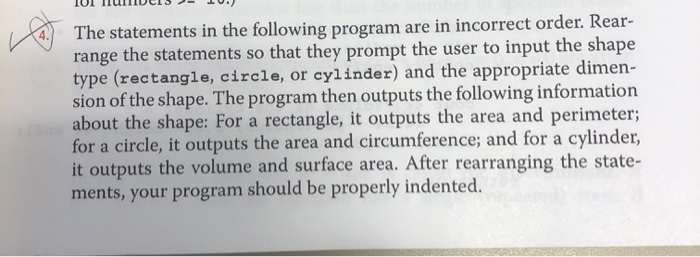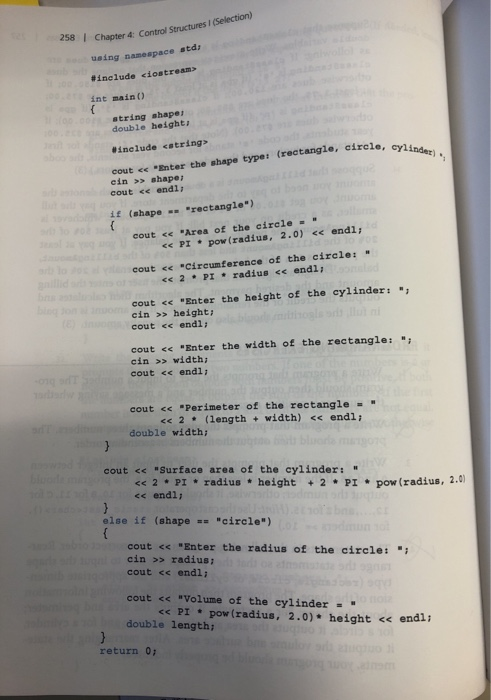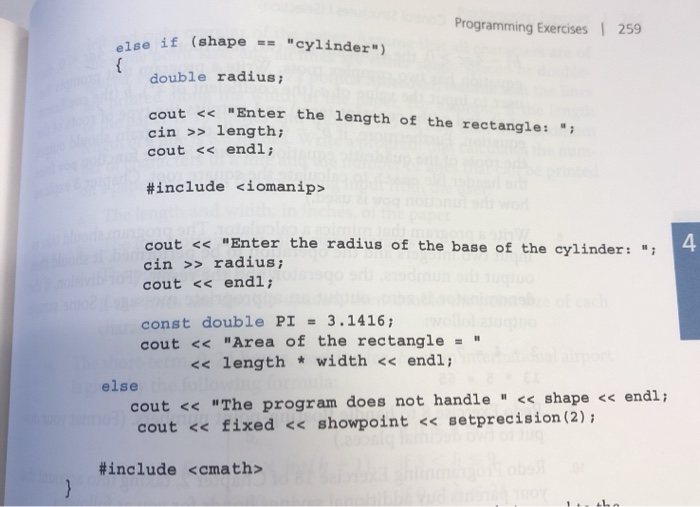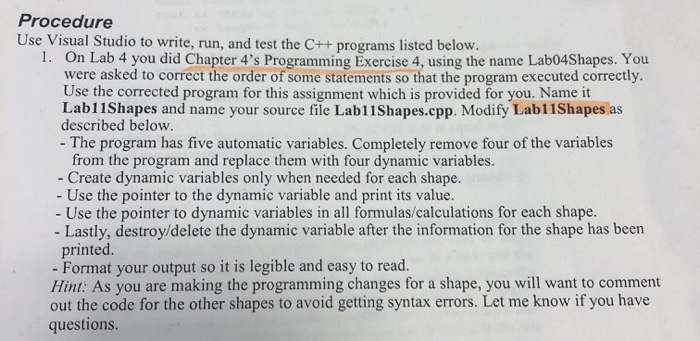# (Solved) : Statements Following Program Incorrect Order Rear Range Statements Prompt User Input Shape Q42699344 . . .The statements in the following program are in incorrect order. Rear- range the statements so that they prompt the user to input the shape type (rectangle, circle, or cylinder) and the appropriate dimen- sion of the shape. The program then outputs the following information about the shape: For a rectangle, it outputs the area and perimeter; for a circle, it outputs the area and circumference; and for a cylinder, it outputs the volume and surface area. After rearranging the state- ments, your program should be properly indented. 258 Chapter 4 Control Structures I (Selection) using namespace std; #include <iostream> int main() string shape double height) he cylinder) #include <string> cout << “Enter the shape type: (rectangle, circle, or cin >> shape; cout << endl; 1 € (shape — “rectangle”) cout << “Area of the circle – << PI – pow (radius, 2.0) << endl; cout << “Circumference of the circle: – << 2 • PI • radius << endl; cout << “Enter the height of the cylinder: “; cin >> height; cout << endl; cout << “Enter the width of the rectangle: “; cin >> width; cout << endl; cout << “Perimeter of the rectangle – – << 2. (length + width) << endl; double width; cout << “Surface area of the cylinder: << 2PI radius * height + 2 . PIpow (radius, 2.0) << endl; else if (shape == “circle”) cout << “Enter the radius of the circle: “; cin >> radius; cout << endl; cout << “Volume of the cylinder – – << PI . pow (radius, 2.0) height << endl; double length; return 0; Programming Exercises | 259 else if (shape == “cylinder) { double radius; cout << “Enter the length of the rectangle: “; cin >> length; cout << endl; #include <iomanip> cout << “Enter the radius of the base of the cylinder: “; cin >> radius; cout << endl; const double PI = 3.1416; cout << “Area of the rectangle -” << length * width << endl; endi. else cout << “The program does not handle ” << shape << endl; cout << fixed << showpoint << setprecision (2); #include <cmath> 11 We were unable to transcribe this imageShow transcribed image text The statements in the following program are in incorrect order. Rear- range the statements so that they prompt the user to input the shape type (rectangle, circle, or cylinder) and the appropriate dimen- sion of the shape. The program then outputs the following information about the shape: For a rectangle, it outputs the area and perimeter; for a circle, it outputs the area and circumference; and for a cylinder, it outputs the volume and surface area. After rearranging the state- ments, your program should be properly indented.
258 Chapter 4 Control Structures I (Selection) using namespace std; #include int main() string shape double height) he cylinder) #include cout shape; cout

Answer to The statements in the following program are in incorrect order. Rear- range the statements so that they prompt the user …

We are the best freelance writing portal. Looking for online writing, editing or proofreading jobs? We have plenty of writing assignments to handle.